# Discrete Probability Distributions Flashcards

Discrete Probability Distributions Flashcards
1/23 (missed) 0 0
Create Your Account To Continue Studying

As a member, you'll also get unlimited access to over 79,000 lessons in math, English, science, history, and more. Plus, get practice tests, quizzes, and personalized coaching to help you succeed.

Try it risk-free for 30 days. Cancel anytime
In a game of blackjack, a person is dealt 2 cards: a 4 and a 9. Assuming aces count as 1, find the probability that the next card dealt makes the blackjack total greater than 21.

Current total: 13

13 + ace through 8 ≤ 21 (31 total cards because one 4 was already used: 31/50 chance)

Any other card: 19/50 = 0.38 = 38% chance

Got it
In a game of blackjack, a person is dealt 2 cards: a 9 and a 10. Assuming aces count as 1, find the probability that the next card dealt makes the blackjack total greater than 21.

Current total: 9 + 10 = 19

19 + ace (1) or 19 + 2 ≤ 21

So, any ace or 2 would give a total of 21 or less. (8 total cards: 8/50 chance)

Any other card: 42/50 = 0.84 = 84% chance

Got it
Find the probability of being dealt two jacks in a row from a standard deck of 52 cards.

Number of jacks in the deck: 4

Probability of first jack: 4/52

Probability of second jack: 3/51

(4/52) * (3/51) = 12/2652 = 1/221

Got it
Find the probability that 0 ≤ X < 2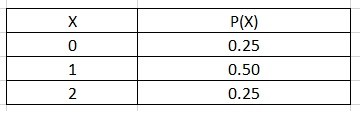0.25 + 0.50 = 0.75 or 75%

Got it
Find the probability that X ≤ 20.25 + 0.50 + 0.25 = 1 or 100%

Got it
Find the probability that X = 20.25 or 25%

Got it
Find the probability of winning \$2 on a roll of two dice if you lose \$5 for rolling a 4, 5, or 6, and win \$2 for rolling anything else.

Roll a 4: 1-3, 3-1, 2-2 (3); Roll a 5: 1-4, 4-1, 2-3, 3-2 (4); Roll a 6: 1-5, 5-1, 2-4, 4-2, 3-3 (5)

(3 + 4 + 5) / 36 = 12/36 chance of losing \$5

36 - 12 = 24/36 = 0.67 = 67% chance of winning \$2

Got it
Find the expected value (the amount of money you expect to win per roll) when rolling 2 dice, losing \$2 for rolling a 4 or 5, winning \$1 for rolling a 2 or 3, and winning \$3 for any other number.

-\$2(7/36) + \$1(3/36) + \$3(26/36) = \$1.86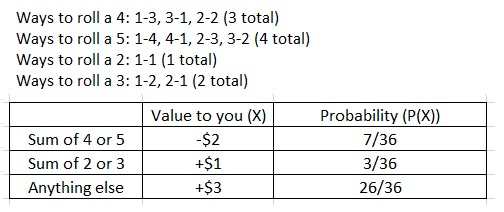Got it
Find the expected value (the amount of money you are expected to win or lose per roll) when rolling two dice, losing \$2 for rolling a 3 or 4, and winning \$3 for any other number.

E(X) = -\$2(5/36) + \$3(31/36) = \$2.31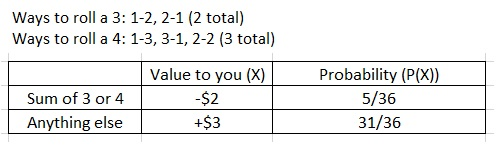Got it
Find the expected value of the random variable X: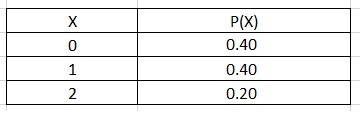E(X) = sum of X * P(X) = (0 * 0.4) + (1 * 0.4) + (2 * 0.2) = 0.8

Got it
Probability Distribution Function or P(X)

This is used to assign a probability to all possible values of X. It is always a number between 0 and 1.

Got it

or choose a specific lesson: See all lessons in this chapter
23 cards in set

## Flashcard Content Overview

What is the relationship between playing cards, flipping a coin, and rolling dice? The probability of their outcomes can be described by discrete probability distribution. Discrete refers to the fact that when rolling dice, for example, you will be dealing with the probability of rolling exact numbers. The flashcards in this set will help you review the various formulas required to solve problems involving discrete probability distribution.

Front
Back
Probability Distribution Function or P(X)

This is used to assign a probability to all possible values of X. It is always a number between 0 and 1.

Find the expected value of the random variable X:E(X) = sum of X * P(X) = (0 * 0.4) + (1 * 0.4) + (2 * 0.2) = 0.8

Find the expected value (the amount of money you are expected to win or lose per roll) when rolling two dice, losing \$2 for rolling a 3 or 4, and winning \$3 for any other number.

E(X) = -\$2(5/36) + \$3(31/36) = \$2.31Find the expected value (the amount of money you expect to win per roll) when rolling 2 dice, losing \$2 for rolling a 4 or 5, winning \$1 for rolling a 2 or 3, and winning \$3 for any other number.

-\$2(7/36) + \$1(3/36) + \$3(26/36) = \$1.86Find the probability of winning \$2 on a roll of two dice if you lose \$5 for rolling a 4, 5, or 6, and win \$2 for rolling anything else.

Roll a 4: 1-3, 3-1, 2-2 (3); Roll a 5: 1-4, 4-1, 2-3, 3-2 (4); Roll a 6: 1-5, 5-1, 2-4, 4-2, 3-3 (5)

(3 + 4 + 5) / 36 = 12/36 chance of losing \$5

36 - 12 = 24/36 = 0.67 = 67% chance of winning \$2

Find the probability that X = 20.25 or 25%

Find the probability that X ≤ 20.25 + 0.50 + 0.25 = 1 or 100%

Find the probability that 0 ≤ X < 20.25 + 0.50 = 0.75 or 75%

Find the probability of being dealt two jacks in a row from a standard deck of 52 cards.

Number of jacks in the deck: 4

Probability of first jack: 4/52

Probability of second jack: 3/51

(4/52) * (3/51) = 12/2652 = 1/221

In a game of blackjack, a person is dealt 2 cards: a 9 and a 10. Assuming aces count as 1, find the probability that the next card dealt makes the blackjack total greater than 21.

Current total: 9 + 10 = 19

19 + ace (1) or 19 + 2 ≤ 21

So, any ace or 2 would give a total of 21 or less. (8 total cards: 8/50 chance)

Any other card: 42/50 = 0.84 = 84% chance

In a game of blackjack, a person is dealt 2 cards: a 4 and a 9. Assuming aces count as 1, find the probability that the next card dealt makes the blackjack total greater than 21.

Current total: 13

13 + ace through 8 ≤ 21 (31 total cards because one 4 was already used: 31/50 chance)

Any other card: 19/50 = 0.38 = 38% chance

Find the probability of being dealt a five-card hand containing 4 jacks from a standard 52-card deck.

Favorable outcomes:

4 jacks in deck = 4! / 4!(4 - 4)! = 1

1 card out of 48 remaining cards = 48! / 1!(48 - 1)! = 48

1*48 = 48

Possible outcomes: 2,598,960

Probability: 48/2,598,960 = 1/54,145

Find the probability of being dealt a five-card hand containing 5 red cards from a standard 52-card deck.

5 red cards out of 26 red cards in deck = 26! / 5!(26 - 5)! = 65,780

Possible outcomes: 2,598,960

Probability: 65,780/2,598,960 = 0.0253

Find the probability of being dealt a five-card hand containing at least 2 kings.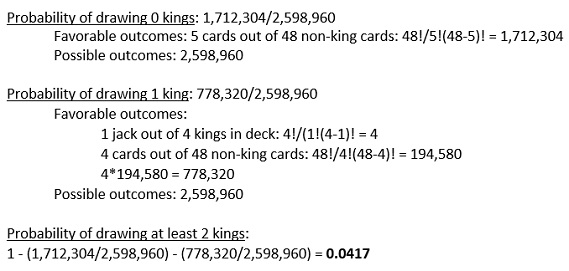Find the probability of being dealt a five-card hand containing at least 3 jacks.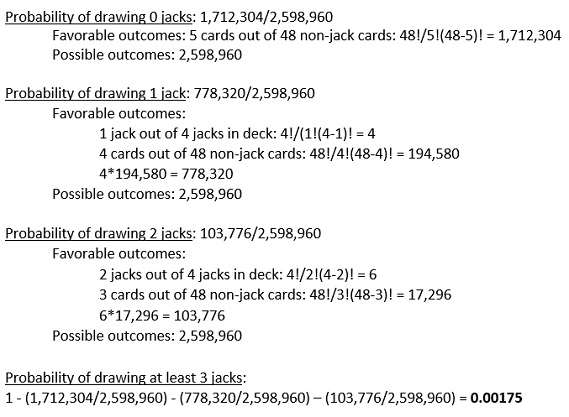Characteristics of Binomial Experiments

1. The outcomes must be independent, so the probability (P) of each trial must be the same.

2. There must be only two possible outcomes.

3. There must be a fixed number of trials (n).

Binomial Probability Equation

B(x; n, P) = nCx * Px * (1 - P)n - x

nCx = combination of n trials with x successes

x = number of successes

n = number of trials

P = probability of success on individual trial

Using the binomial probability formula, find the probability that 1 person voted out of 5 if each person had a 50% chance of voting.

Probability of zero voting:

x=0, n=5, P=0.50

nCx = 5! / 0!(5-0)! = 1

B(x; n, P) = nCx * Px * (1-P)n-x = 1 * (0.50)0 * (1 - 0.50)5-0 = 0.0313

1 - 0.0313 = 0.9687 = 96.9% chance that 1 person out of 5 voted

Using the binomial probability formula, find the probability that 0 or 1 people voted out of 5 if each person had a 50% chance of voting.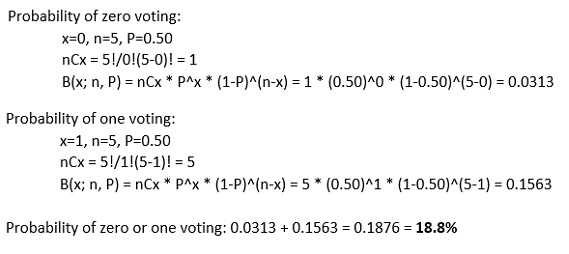Using the binomial probability formula, find the probability that 2 people voted out of 5 if each person had a 50% chance of voting.

x = 2, n = 5, P = 0.50

nCx = 5! / 2!(5 - 2)! = 10

B(x; n, P) = nCx * Px * (1 - P)n - x = 10 * (0.50)2 * (1 - 0.50)5 - 2 = 0.3125 = 31.2%

Find the probability of winning 4 out of 5 games of craps if there is a 0.493 chance of winning each game.

x = 4, n = 5, P = 0.493

nCx = 5! / 4!(5 - 4)! = 5

B(x; n, P) = nCx * Px * (1 - P)n - x = 5 * (0.493)4 * (1 - 0.493)5 - 4 = 0.1497 = 15%

Using the binomial probability formula, find the probability that x = 3 if P = 0.30 and n = 4.

x = 3, n = 4, P = 0.30

nCx = 4! / 3!(4 - 3)! = 4

B(x; n, P) = nCx * Px * (1 - P)n - x = 4 * (0.30)3 * (1 - 0.30)4 - 3 = 0.0756 = 7.6%

Binomial Experiment

An experiment in which there are only two discrete outcomes: success or failure. The outcomes of individual trials are independent of one another.

To unlock this flashcard set you must be a Study.com Member.